# Chapter 9 Chemical Bonding I The Lewis Model

• Slides: 90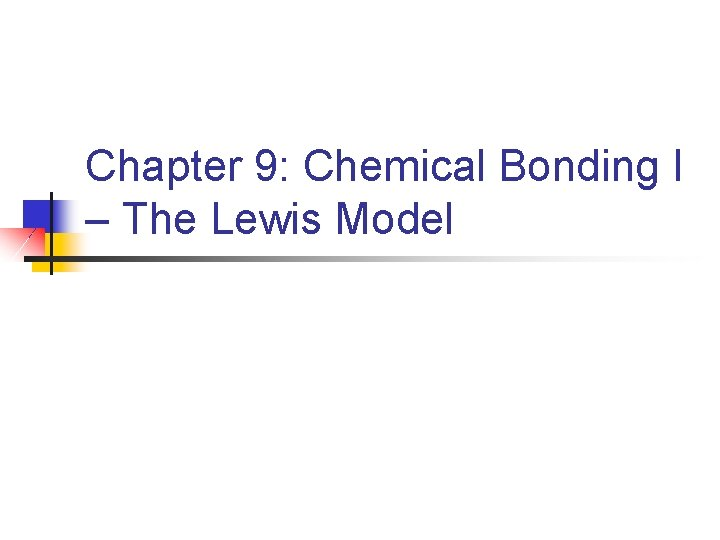Chapter 9: Chemical Bonding I – The Lewis Model Homework: 1, 8, 11, 12, 13, 16, 18, 19, 20, 22, 24, 25, 30, 31, 32, 35, 37, 39, 40, 42, 43, 45, 47, 49, 50, 53, 54, 57, 59, 61, 62, 65, 66, 71, 72, 97, 1049. 2 – Types of Chemical Bonds When two atoms or ions are strongly attracted, there is a chemical bond. Three types of chemical bonds n n 1. Ionic bond n n 2. Covalent bond n n 3. Electrostatic forces holding together ions of opposite charge Generally between metals and nonmetals Sharing of electrons Generally between two nonmetals Metallic bond n n Between two or more metals Bonding electrons are free to move around9. 3 and 9. 4: Lewis Symbols, Lattice Energy and Ionic Bonds n A way of showing the valence electrons in an atom n n Used to track the electrons during bond formation The Lewis symbol for an element consists of n n chemical symbol for the element a dot for each valence electronn The dots are placed on the four sides of the atomic symbol n n Top, bottom, left and right Each side can have 2 electrons (dots) All four sides are equivalent The number of dots for any representative element = group #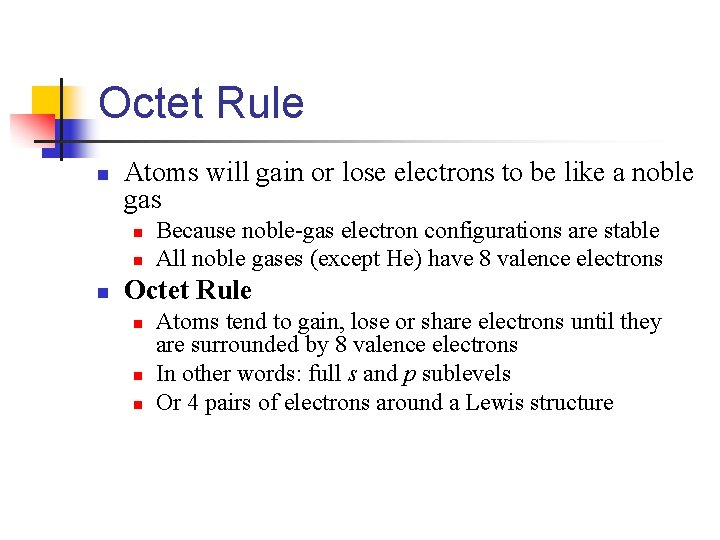Octet Rule n Atoms will gain or lose electrons to be like a noble gas n n n Because noble-gas electron configurations are stable All noble gases (except He) have 8 valence electrons Octet Rule n n n Atoms tend to gain, lose or share electrons until they are surrounded by 8 valence electrons In other words: full s and p sublevels Or 4 pairs of electrons around a Lewis structure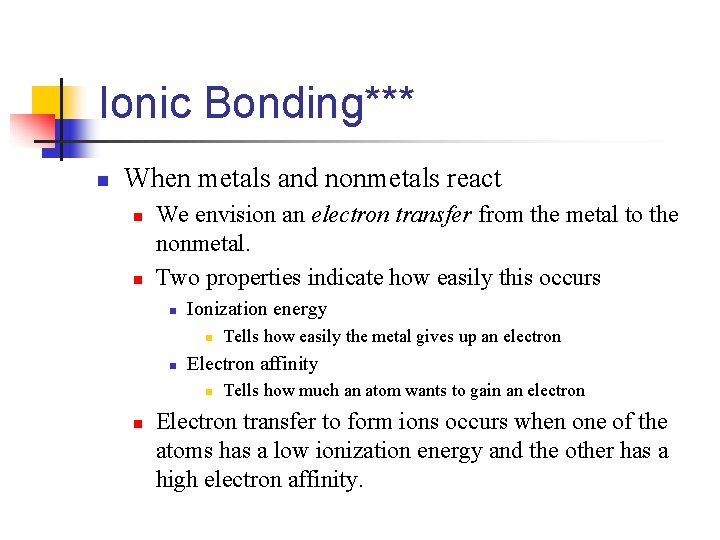Ionic Bonding*** n When metals and nonmetals react n n We envision an electron transfer from the metal to the nonmetal. Two properties indicate how easily this occurs n Ionization energy n n Electron affinity n n Tells how easily the metal gives up an electron Tells how much an atom wants to gain an electron Electron transfer to form ions occurs when one of the atoms has a low ionization energy and the other has a high electron affinity.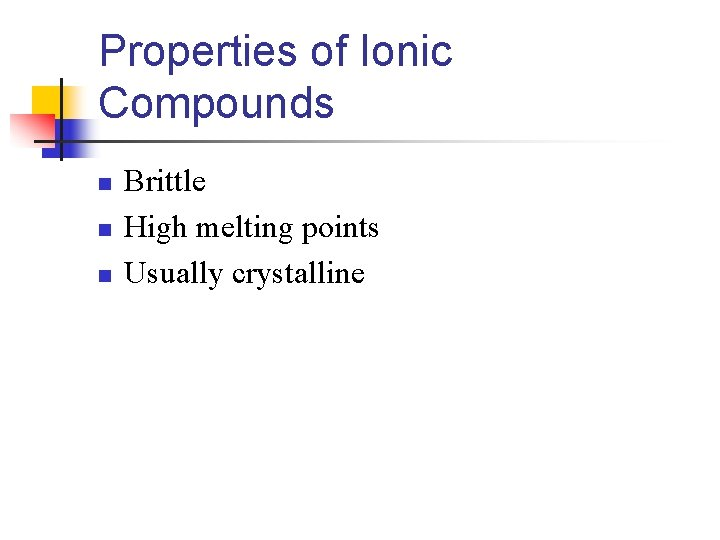Properties of Ionic Compounds n n n Brittle High melting points Usually crystalline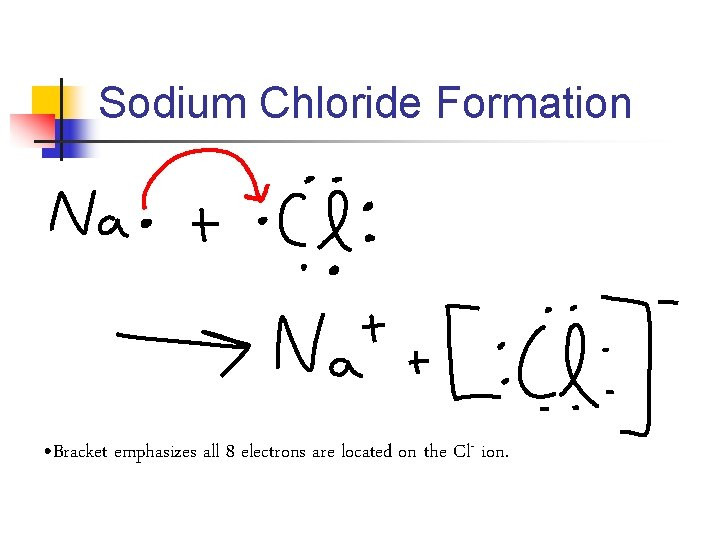Sodium Chloride Formation • Bracket emphasizes all 8 electrons are located on the Cl- ion.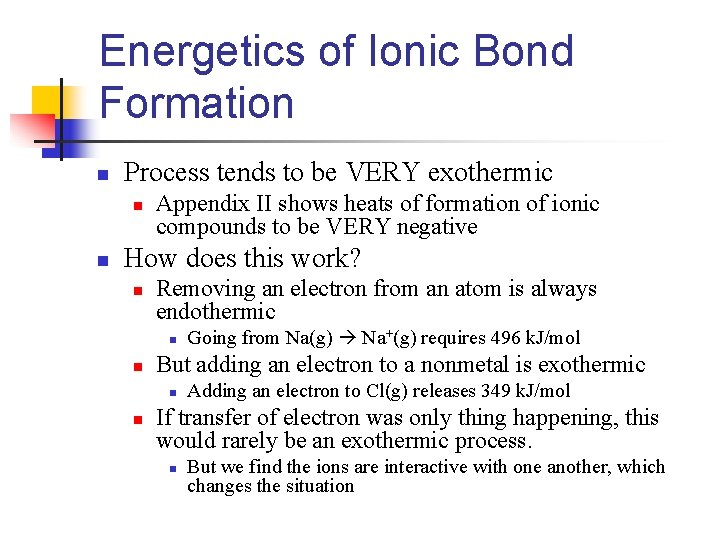Energetics of Ionic Bond Formation n Process tends to be VERY exothermic n n Appendix II shows heats of formation of ionic compounds to be VERY negative How does this work? n Removing an electron from an atom is always endothermic n n But adding an electron to a nonmetal is exothermic n n Going from Na(g) Na+(g) requires 496 k. J/mol Adding an electron to Cl(g) releases 349 k. J/mol If transfer of electron was only thing happening, this would rarely be an exothermic process. n But we find the ions are interactive with one another, which changes the situationn Ionic compounds are stable primarily because of the attraction between ions of unlike charge. n n n This attraction drawn ions together This releases energy and causes ions to form a solid array, or lattice A measure of how much stabilization comes from arranging oppositely charged ions in an ionic solid is given by the lattice energy n Lattice energy is also the amount of energy required to completely separate a mole of a solid ionic compound into its gaseous ions.n Na. Cl(s) Na+(g) + Cl-(g) ΔHlattice = +788 k. J/mol n n This is EXTREMELY endothermic BUT n The reverse process - the joining together of Na+(g) and Cl-(g) is therefore EXTREMELY exothermic (ΔH = -788 k. J/mol)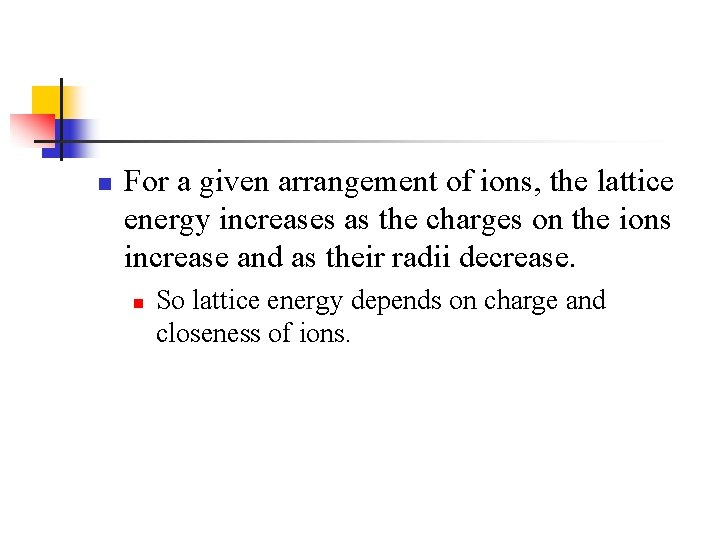n For a given arrangement of ions, the lattice energy increases as the charges on the ions increase and as their radii decrease. n So lattice energy depends on charge and closeness of ions.Example n Which of the following would have the greatest lattice energy? n n Ag. Cl, Cu. O, Cr. N! Why? Cr 3+ and N 3 - have the greatest charges on them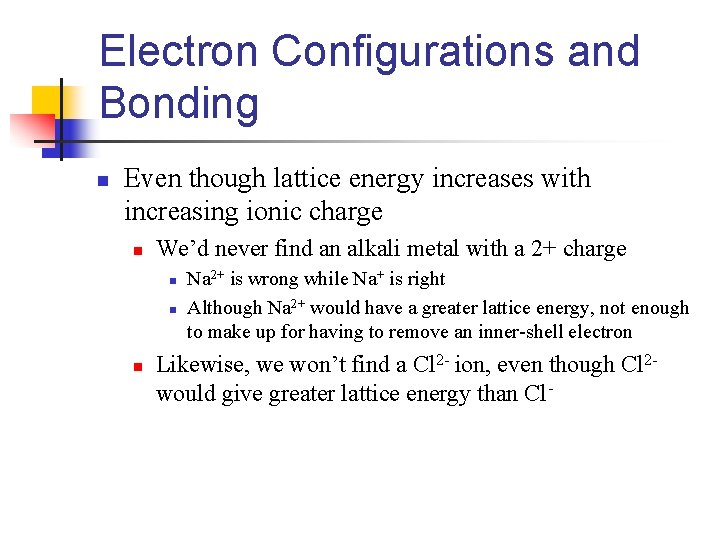Electron Configurations and Bonding n Even though lattice energy increases with increasing ionic charge n We’d never find an alkali metal with a 2+ charge n n n Na 2+ is wrong while Na+ is right Although Na 2+ would have a greater lattice energy, not enough to make up for having to remove an inner-shell electron Likewise, we won’t find a Cl 2 - ion, even though Cl 2 would give greater lattice energy than Cl-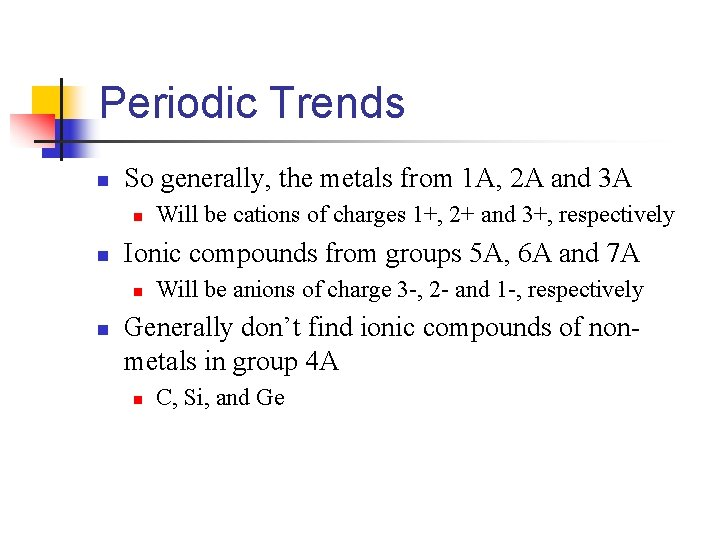Periodic Trends n So generally, the metals from 1 A, 2 A and 3 A n n Ionic compounds from groups 5 A, 6 A and 7 A n n Will be cations of charges 1+, 2+ and 3+, respectively Will be anions of charge 3 -, 2 - and 1 -, respectively Generally don’t find ionic compounds of nonmetals in group 4 A n C, Si, and GeTransition Metal Ions n The lattice energies of ionic compounds usually only large enough to make up for the loss of three electrons n n n 1+, 2+ and 3+ cations only Octet rule broken by most transition metals When forming ions, transition metals lose the valence-shell electrons first, then as many d electrons as are required to reach the charge of the ionIron Example n Fe n n When forming Fe 2+ n n [Ar]3 d 64 s 2 [Ar]3 d 6 When forming Fe 3+ n [Ar]3 d 5Polyatomic Ions n Several cations and many common anions are polyatomic n n n NH 4+ = ammonium CO 32 - = carbonate In these, two or more atoms bound together by mostly covalent bonds. n n They form a stable group that carries a charge Only thing you need to know n Group of atoms as a whole acts as a single charged thing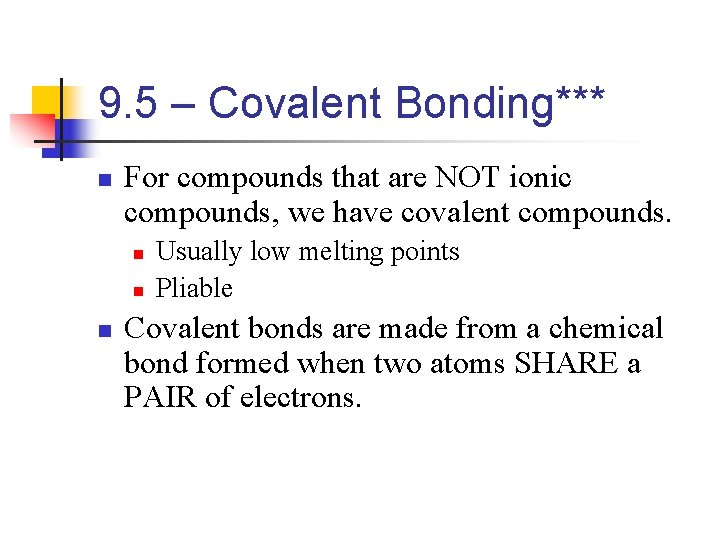9. 5 – Covalent Bonding*** n For compounds that are NOT ionic compounds, we have covalent compounds. n n n Usually low melting points Pliable Covalent bonds are made from a chemical bond formed when two atoms SHARE a PAIR of electrons.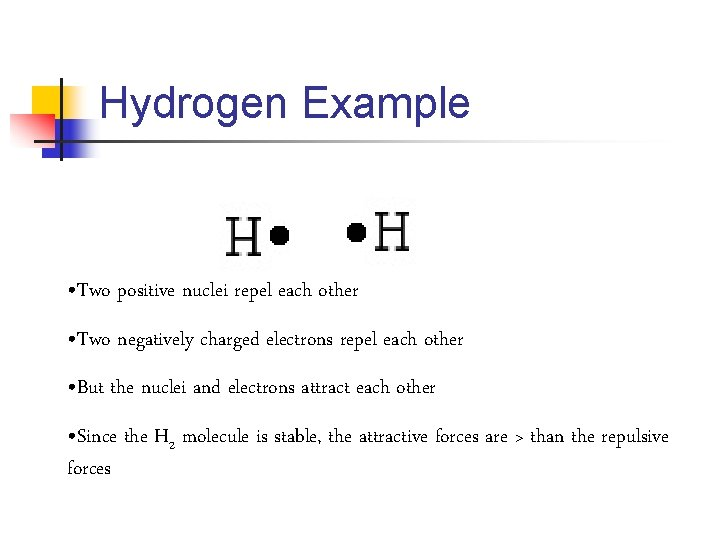Hydrogen Example • Two positive nuclei repel each other • Two negatively charged electrons repel each other • But the nuclei and electrons attract each other • Since the H 2 molecule is stable, the attractive forces are > than the repulsive forcesn n So the nuclei are each attracted to the negative charge from the shared electrons between them. Simply, the shared pair of electrons in any covalent bond acts like a “glue” to bind the atoms together.Lewis Structures n Octet rule!!!!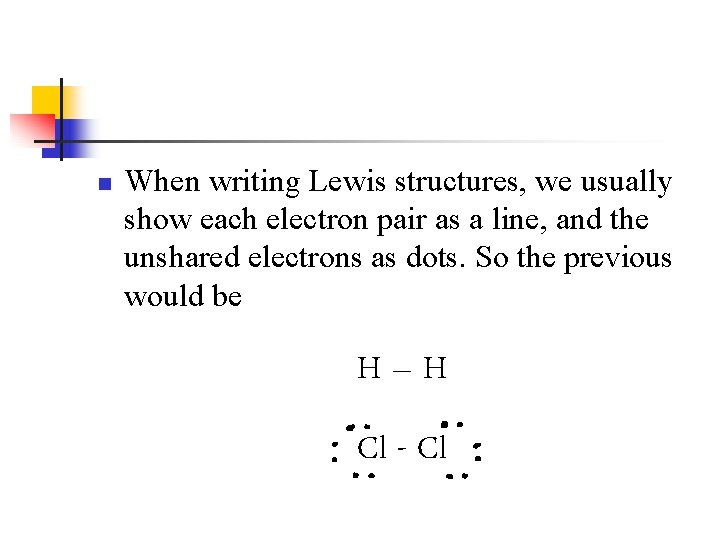n When writing Lewis structures, we usually show each electron pair as a line, and the unshared electrons as dots. So the previous would be H–H Cl - Cl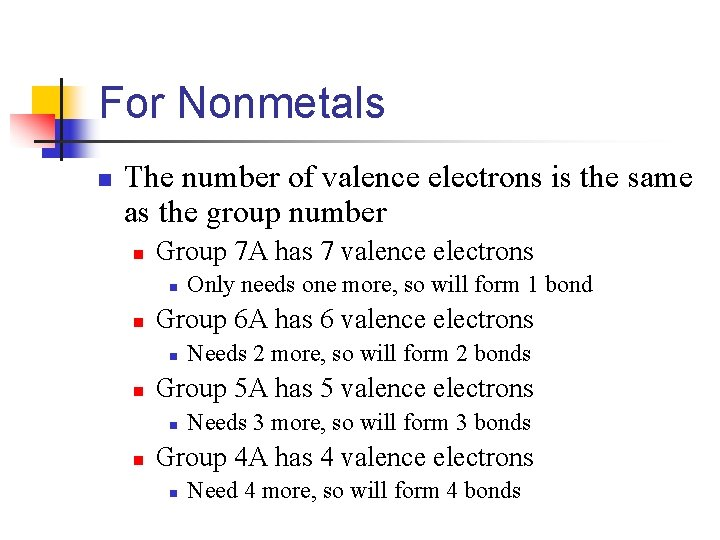For Nonmetals n The number of valence electrons is the same as the group number n Group 7 A has 7 valence electrons n n Group 6 A has 6 valence electrons n n Needs 2 more, so will form 2 bonds Group 5 A has 5 valence electrons n n Only needs one more, so will form 1 bond Needs 3 more, so will form 3 bonds Group 4 A has 4 valence electrons n Need 4 more, so will form 4 bonds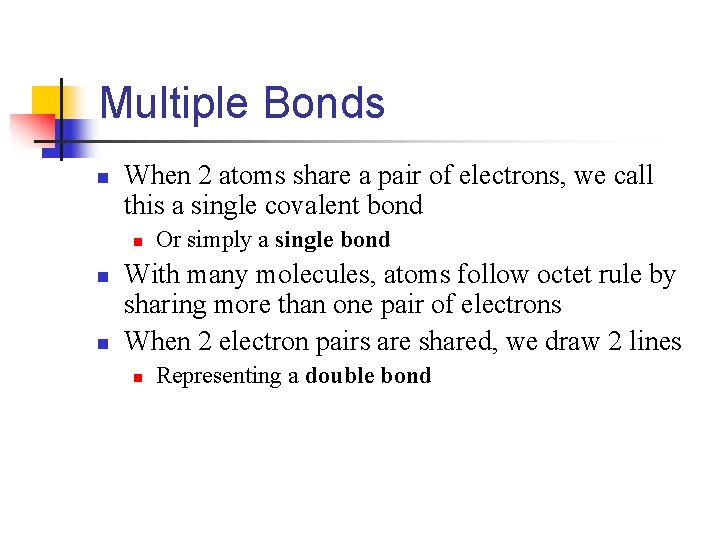Multiple Bonds n When 2 atoms share a pair of electrons, we call this a single covalent bond n n n Or simply a single bond With many molecules, atoms follow octet rule by sharing more than one pair of electrons When 2 electron pairs are shared, we draw 2 lines n Representing a double bond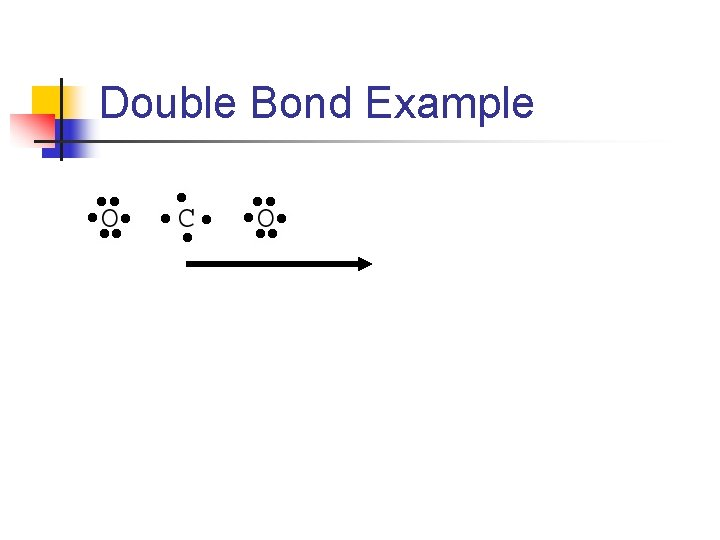Double Bond Example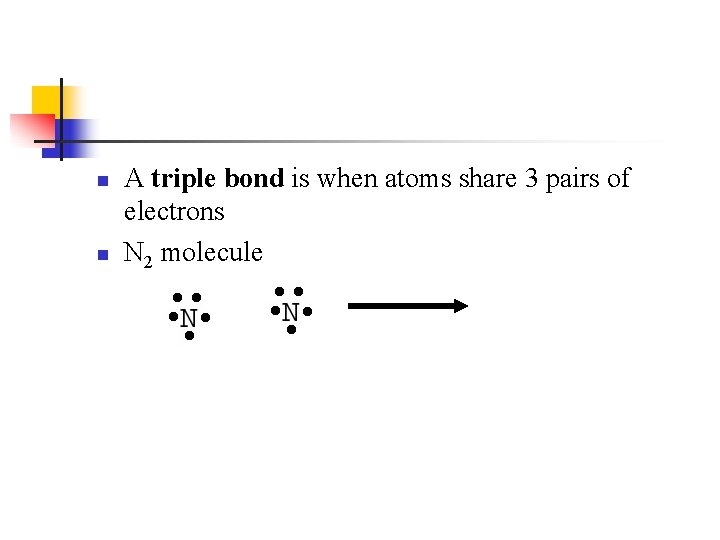n n A triple bond is when atoms share 3 pairs of electrons N 2 molecule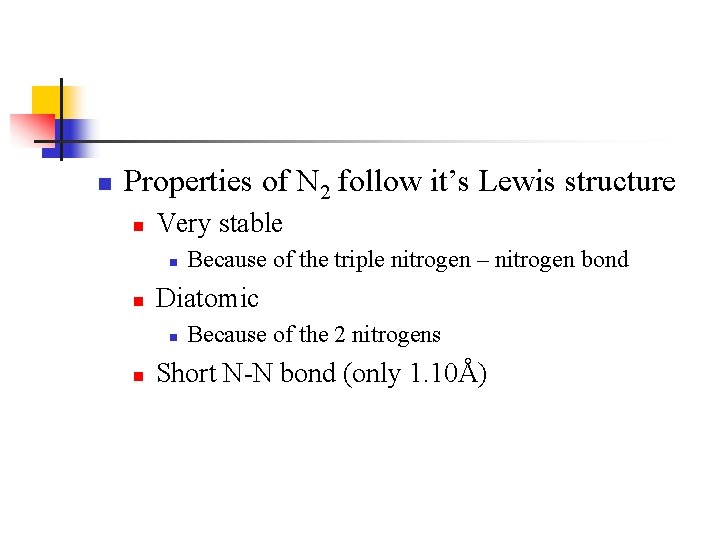n Properties of N 2 follow it’s Lewis structure n Very stable n n Diatomic n n Because of the triple nitrogen – nitrogen bond Because of the 2 nitrogens Short N-N bond (only 1. 10Å)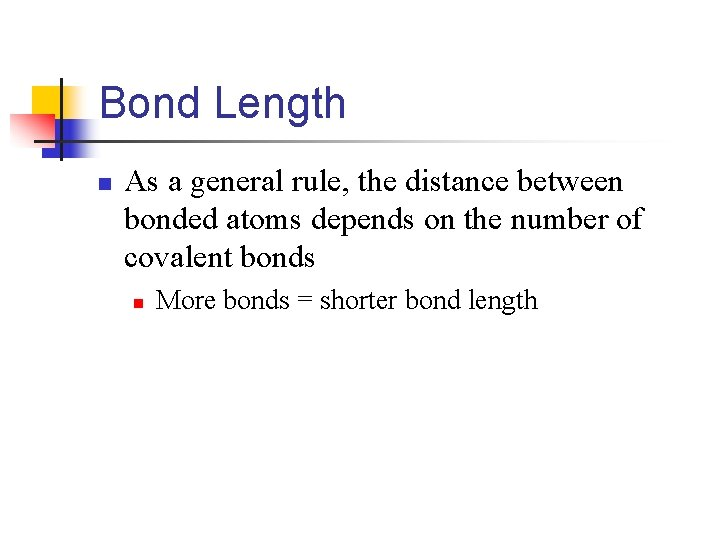Bond Length n As a general rule, the distance between bonded atoms depends on the number of covalent bonds n More bonds = shorter bond length9. 6 – Bond Polarity and Electronegativity**** n n When identical atoms bond, the electron pairs are shared equally However, with Na. Cl there is no sharing of electrons n n n Because it is composed of Na+ and Cl- ions The 3 s electron from Na transferred completely to Cl For covalent substances, the bonds occur somewhere between these extremes.Electronegativity n Electronegativity lets us estimate if a given bond is nonpolar covalent, polar covalent or ionic. n n Electronegativity is the ability of an atom in a compound to attract electrons to itself The greater the atoms electronegativity, the greater its ability to attract electrons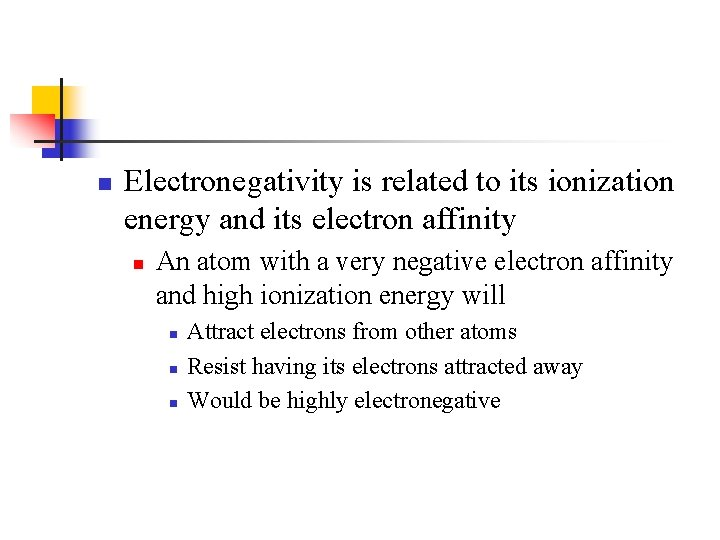n Electronegativity is related to its ionization energy and its electron affinity n An atom with a very negative electron affinity and high ionization energy will n n n Attract electrons from other atoms Resist having its electrons attracted away Would be highly electronegative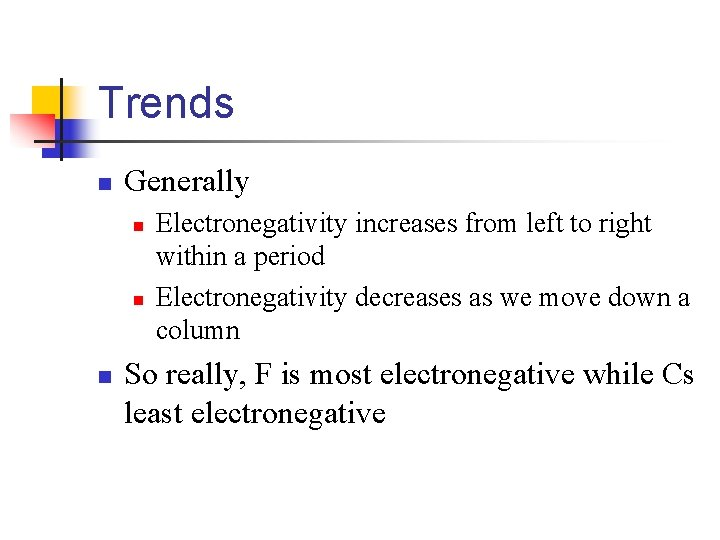Trends n Generally n n n Electronegativity increases from left to right within a period Electronegativity decreases as we move down a column So really, F is most electronegative while Cs least electronegativeBond Polarity n We can use differences in electronegativity between two atoms to estimate the polarity of the bond between them Compound F 2 HF Li. F Electronegativity difference 4. 0 – 4. 0 = 0 4. 0 – 2. 1 = 1. 9 4. 0 – 1. 0 = 3. 0 Type of bond Nonpolar covalent Polar Covalent Ionic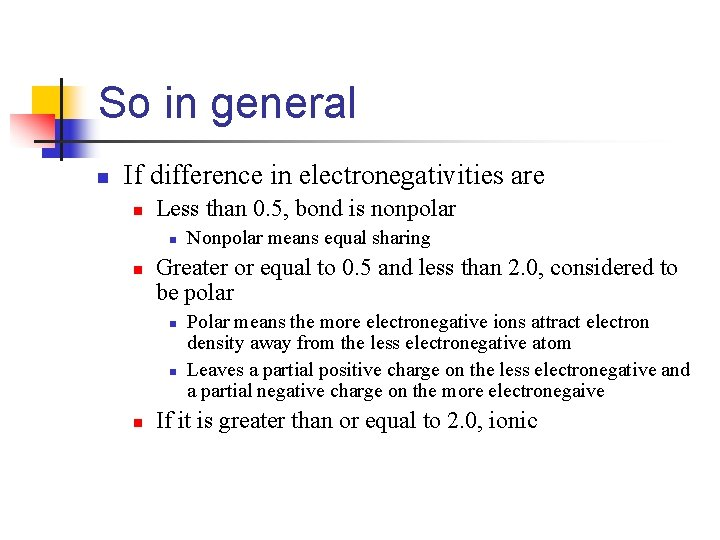So in general n If difference in electronegativities are n Less than 0. 5, bond is nonpolar n n Greater or equal to 0. 5 and less than 2. 0, considered to be polar n n n Nonpolar means equal sharing Polar means the more electronegative ions attract electron density away from the less electronegative atom Leaves a partial positive charge on the less electronegative and a partial negative charge on the more electronegaive If it is greater than or equal to 2. 0, ionicHF Example δ+ δ- H-F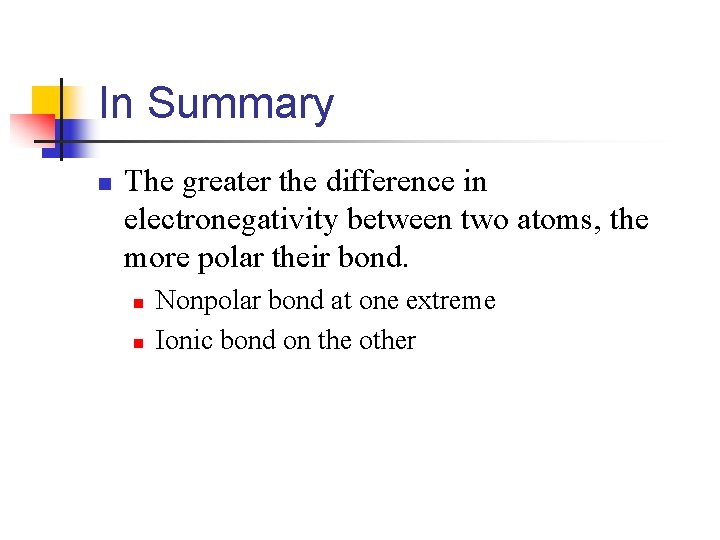In Summary n The greater the difference in electronegativity between two atoms, the more polar their bond. n n Nonpolar bond at one extreme Ionic bond on the other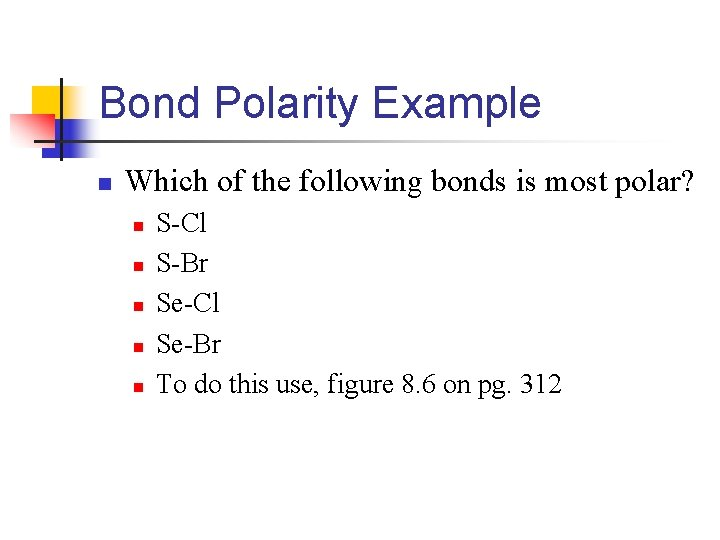Bond Polarity Example n Which of the following bonds is most polar? n n n S-Cl S-Br Se-Cl Se-Br To do this use, figure 8. 6 on pg. 312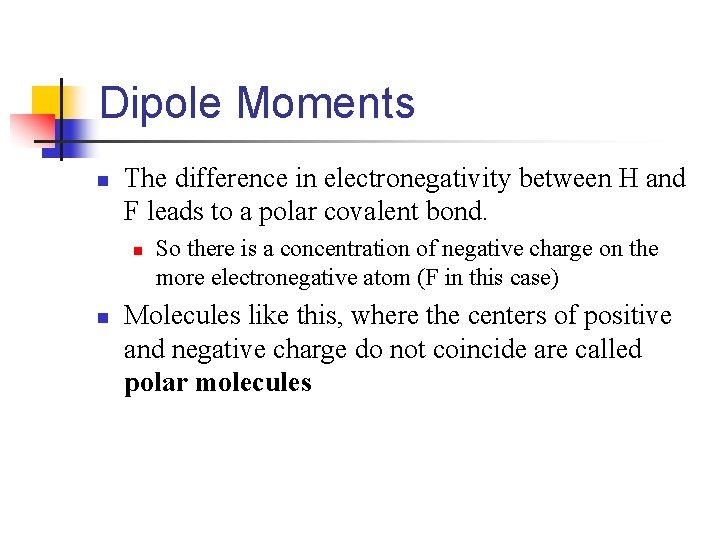Dipole Moments n The difference in electronegativity between H and F leads to a polar covalent bond. n n So there is a concentration of negative charge on the more electronegative atom (F in this case) Molecules like this, where the centers of positive and negative charge do not coincide are called polar molecules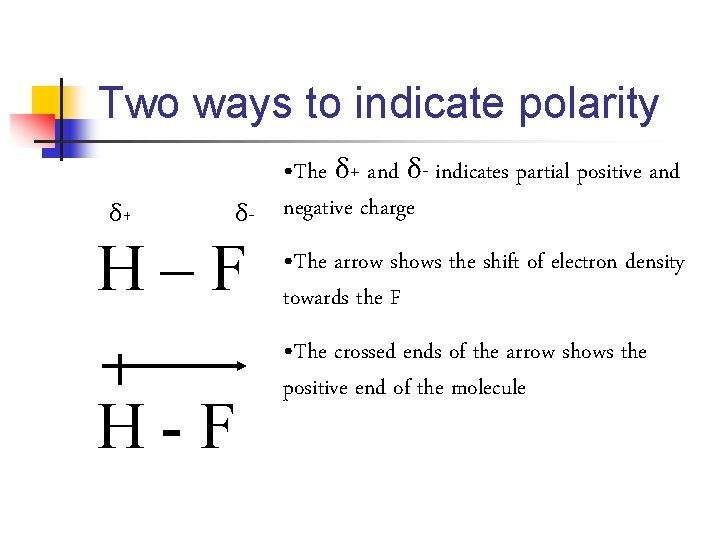Two ways to indicate polarity δ+ • The δ+ and δ- indicates partial positive and δ- negative charge H–F H-F • The arrow shows the shift of electron density towards the F • The crossed ends of the arrow shows the positive end of the molecule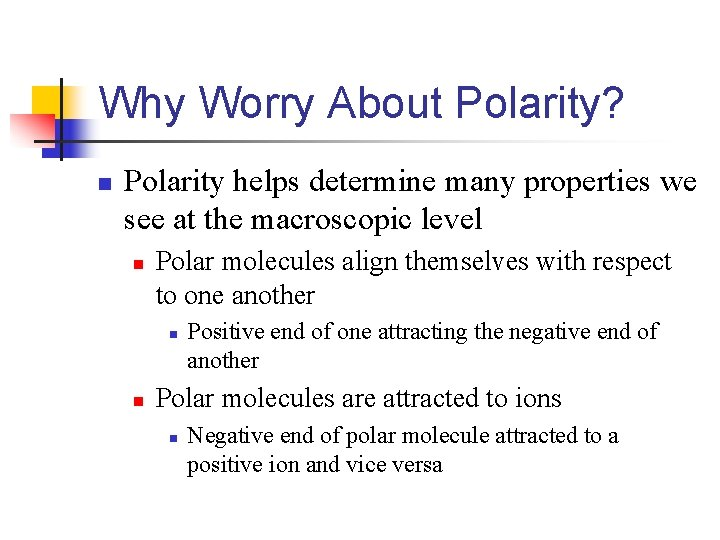Why Worry About Polarity? n Polarity helps determine many properties we see at the macroscopic level n Polar molecules align themselves with respect to one another n n Positive end of one attracting the negative end of another Polar molecules are attracted to ions n Negative end of polar molecule attracted to a positive ion and vice versaDipole Moment n Whenever two electrical charges of equal magnitude (amount) but opposite sign are separated by a distance a dipole is created. n n The quantitative measure of the amount of the dipole is called its dipole moment (μ) If two equal and opposite charges Q+ and Q- are separated by distance r, the magnitude of the dipole moment is μ = Qrn The dipole moment increases as n n n The magnitude of the charges increases The distance between the charges increases For nonpolar molecules n n The dipole moment is zero Because there is no charge separationBond Length n As electronegativity differences decreases the bond length increasesBond Types and Nomenclature n We’ve seen ways to name ionic and molecular compounds n But when we get metals with high oxidation numbers (like Ti 4+) the names blur a little n n n Ti. O 2 Called either titanium (IV) oxide or titanium dioxide These high oxidation number metal-nonmetal compounds actually contains covalent bonds, so is really a molecular compound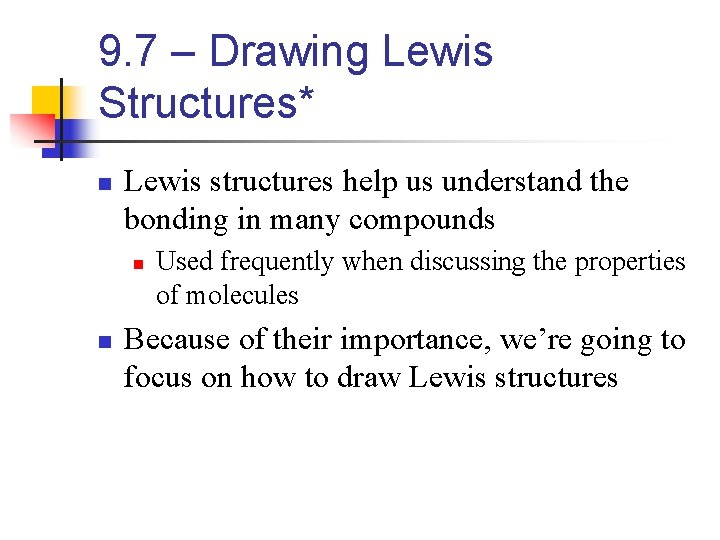9. 7 – Drawing Lewis Structures* n Lewis structures help us understand the bonding in many compounds n n Used frequently when discussing the properties of molecules Because of their importance, we’re going to focus on how to draw Lewis structures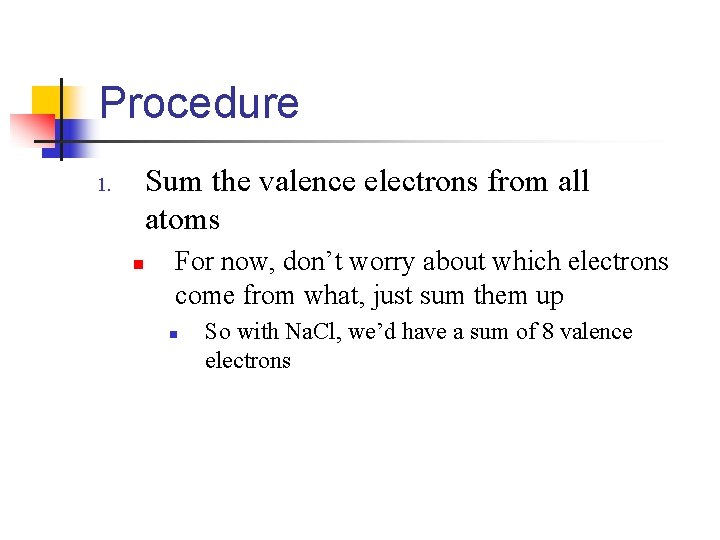Procedure Sum the valence electrons from all atoms 1. n For now, don’t worry about which electrons come from what, just sum them up n So with Na. Cl, we’d have a sum of 8 valence electrons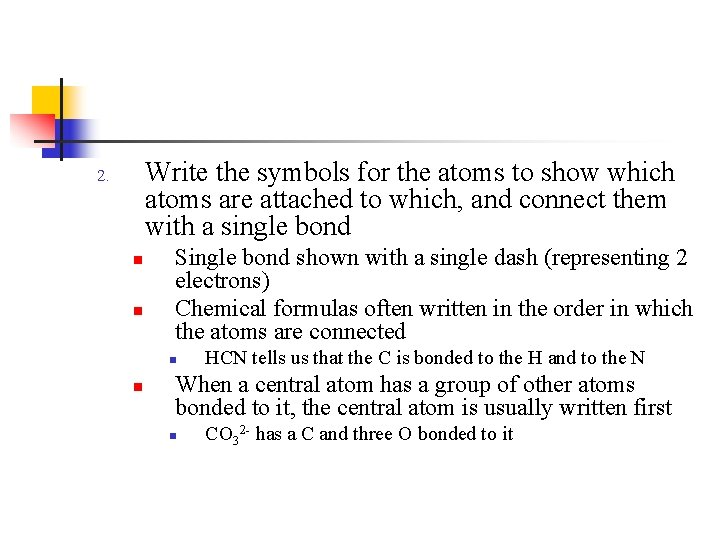Write the symbols for the atoms to show which atoms are attached to which, and connect them with a single bond 2. n n Single bond shown with a single dash (representing 2 electrons) Chemical formulas often written in the order in which the atoms are connected n n HCN tells us that the C is bonded to the H and to the N When a central atom has a group of other atoms bonded to it, the central atom is usually written first n CO 32 - has a C and three O bonded to it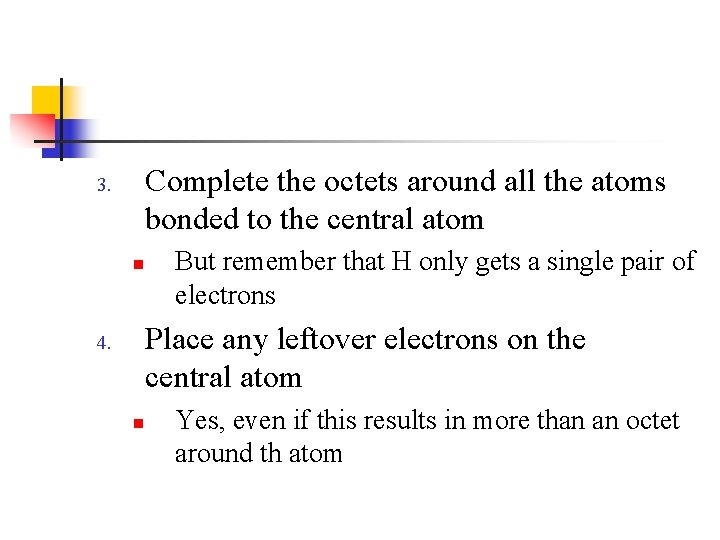Complete the octets around all the atoms bonded to the central atom 3. n But remember that H only gets a single pair of electrons Place any leftover electrons on the central atom 4. n Yes, even if this results in more than an octet around th atom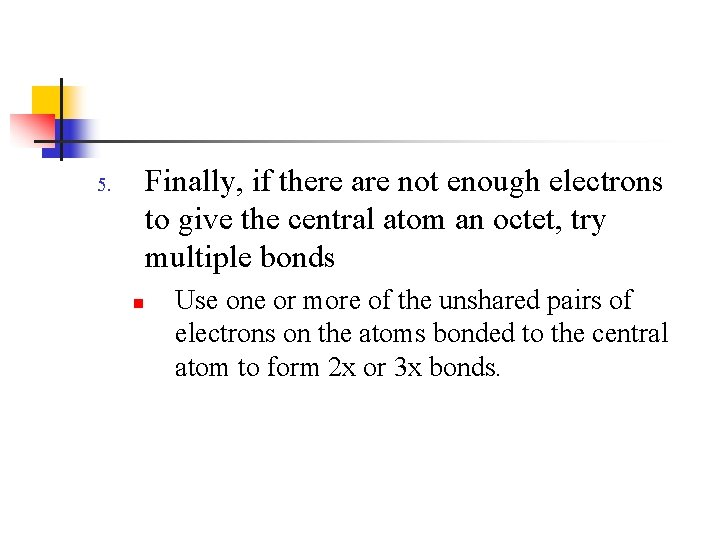Finally, if there are not enough electrons to give the central atom an octet, try multiple bonds 5. n Use one or more of the unshared pairs of electrons on the atoms bonded to the central atom to form 2 x or 3 x bonds.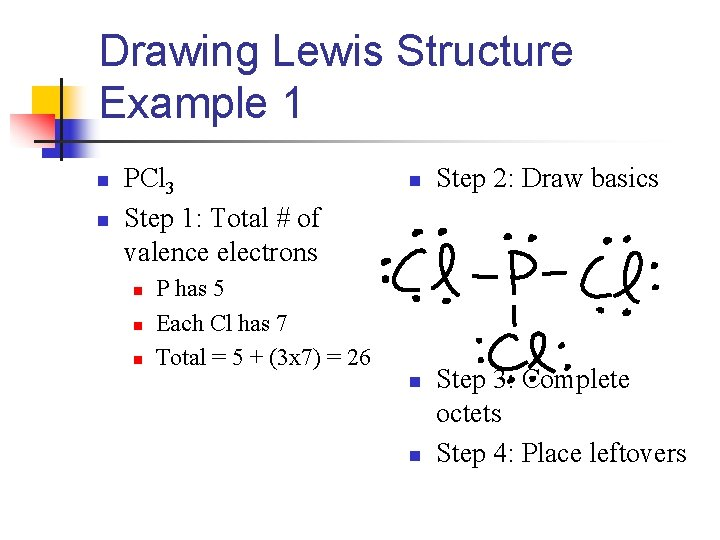Drawing Lewis Structure Example 1 n n PCl 3 Step 1: Total # of valence electrons n n P has 5 Each Cl has 7 Total = 5 + (3 x 7) = 26 n n Step 2: Draw basics Step 3: Complete octets Step 4: Place leftovers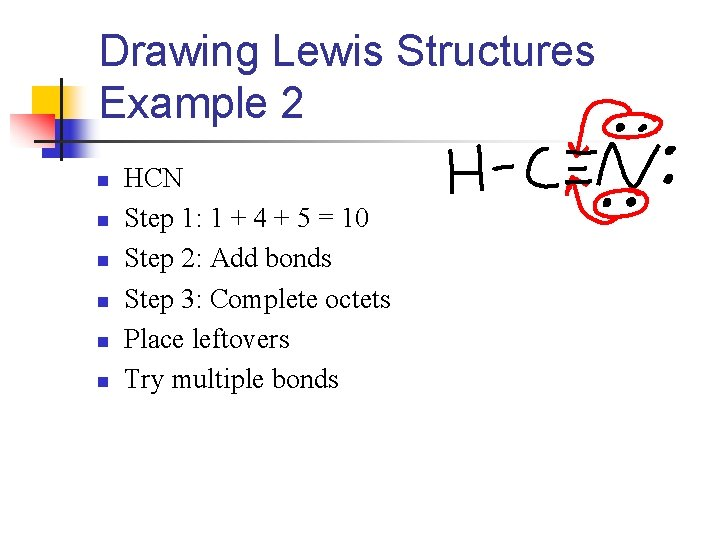Drawing Lewis Structures Example 2 n n n HCN Step 1: 1 + 4 + 5 = 10 Step 2: Add bonds Step 3: Complete octets Place leftovers Try multiple bonds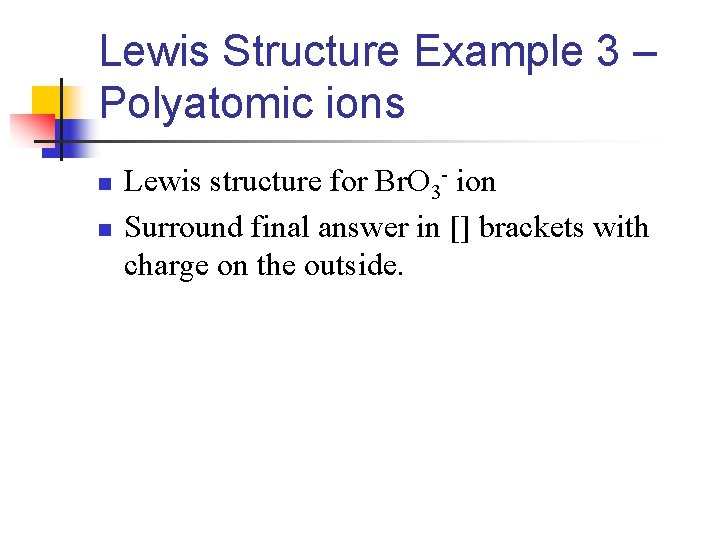Lewis Structure Example 3 – Polyatomic ions n n Lewis structure for Br. O 3 - ion Surround final answer in [] brackets with charge on the outside.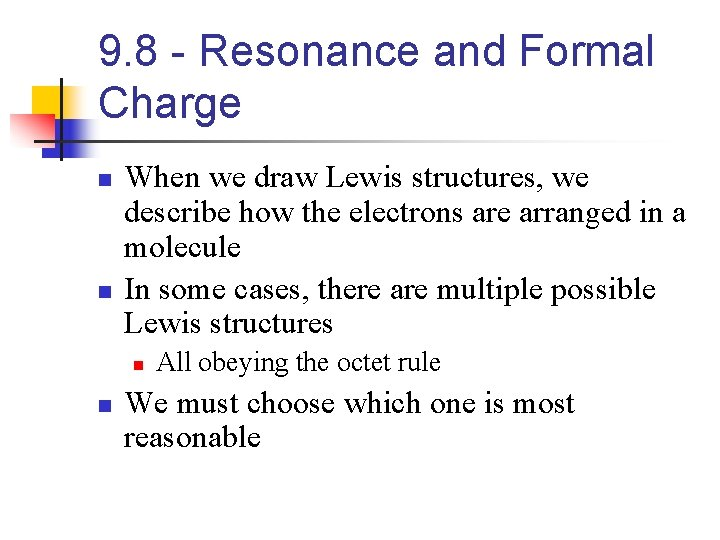9. 8 - Resonance and Formal Charge n n When we draw Lewis structures, we describe how the electrons are arranged in a molecule In some cases, there are multiple possible Lewis structures n n All obeying the octet rule We must choose which one is most reasonable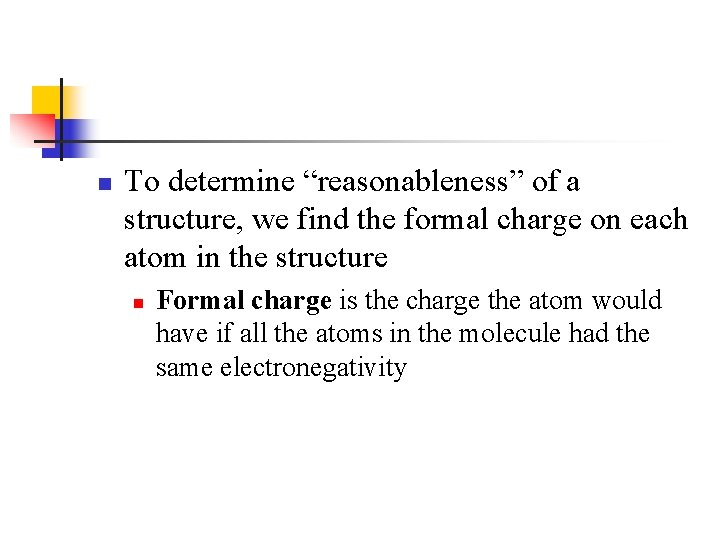n To determine “reasonableness” of a structure, we find the formal charge on each atom in the structure n Formal charge is the charge the atom would have if all the atoms in the molecule had the same electronegativityHow to Calculate Formal Charge We assign the electrons to the atom as follows: n 1. 2. n n All unshared (nonbinding) electrons are assigned to the atom on which they are found For any bond – single, double or triple – half of the bonding electrons are assigned to each atom in the bond The formal charge is then found by subtracting the number of electrons assigned to the atom from the number of valence electrons in the isolated atom Don’t panic, here are some examples…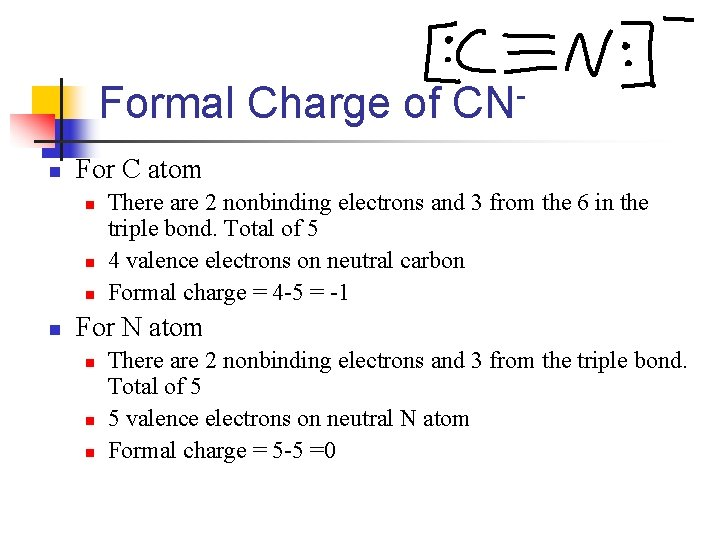Formal Charge of CNn For C atom n n There are 2 nonbinding electrons and 3 from the 6 in the triple bond. Total of 5 4 valence electrons on neutral carbon Formal charge = 4 -5 = -1 For N atom n n n There are 2 nonbinding electrons and 3 from the triple bond. Total of 5 5 valence electrons on neutral N atom Formal charge = 5 -5 =0n n So the formal charges on the atoms in the Lewis structure of CN- are The sum of the formal charges equals the overall charge of the molecule n n Since CN- has a single negative charge, we expect its formal charge to be -1 H 2 O would be expected to have a formal charge of 0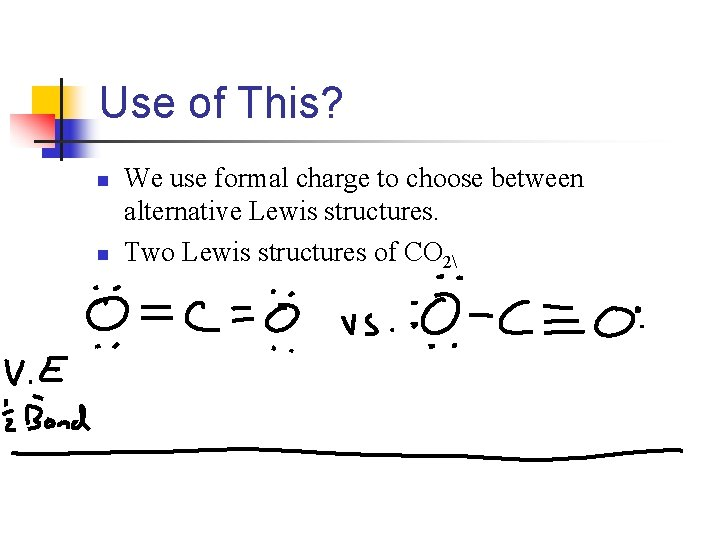Use of This? n n We use formal charge to choose between alternative Lewis structures. Two Lewis structures of CO 2n In both cases, formal charge on the molecule adds up to zero n n Which makes sense, because CO 2 is neutral So which is correct?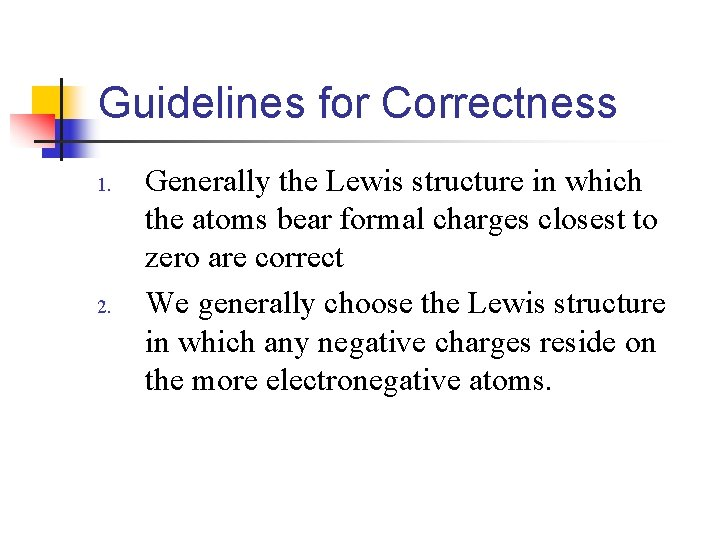Guidelines for Correctness 1. 2. Generally the Lewis structure in which the atoms bear formal charges closest to zero are correct We generally choose the Lewis structure in which any negative charges reside on the more electronegative atoms.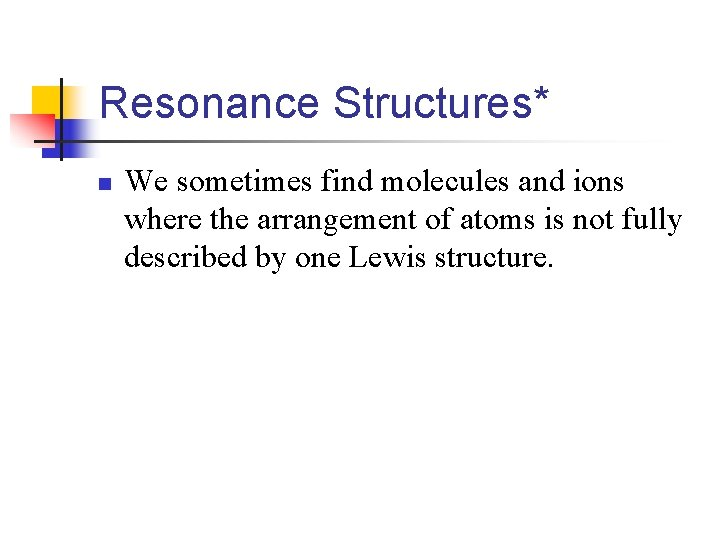Resonance Structures* n We sometimes find molecules and ions where the arrangement of atoms is not fully described by one Lewis structure.Ozone, O 3 n 18 total valence electrons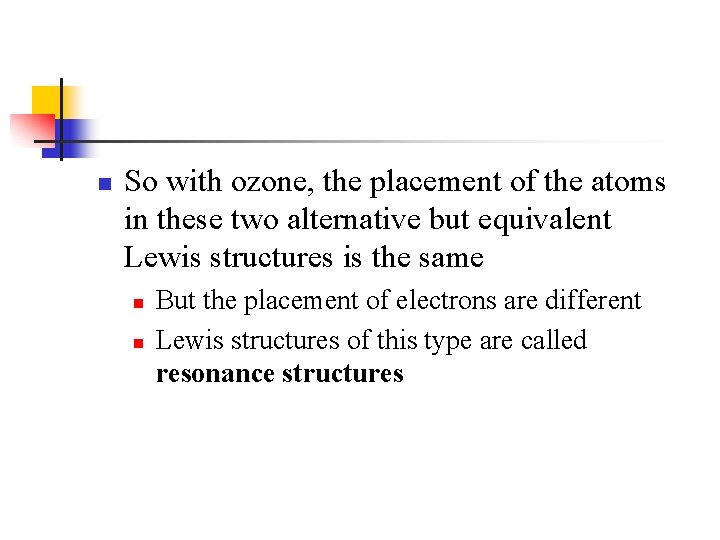n So with ozone, the placement of the atoms in these two alternative but equivalent Lewis structures is the same n n But the placement of electrons are different Lewis structures of this type are called resonance structuresn So to describe O 3 completely n n n Write both Lewis structures and use a double-headed arrow This indicates that the real molecule is described by an average of the 2 resonance structures The true arrangement of the electrons is considered a “blend” of the two (or more) Lewis structures n So with ozone, the two O-O bonds are somewhere between the lengths of a single bond a double bond.n When writing resonance structures you must remember n n The arrangement of the atoms are the same for each equivalent configuration Only where the electrons are placed is different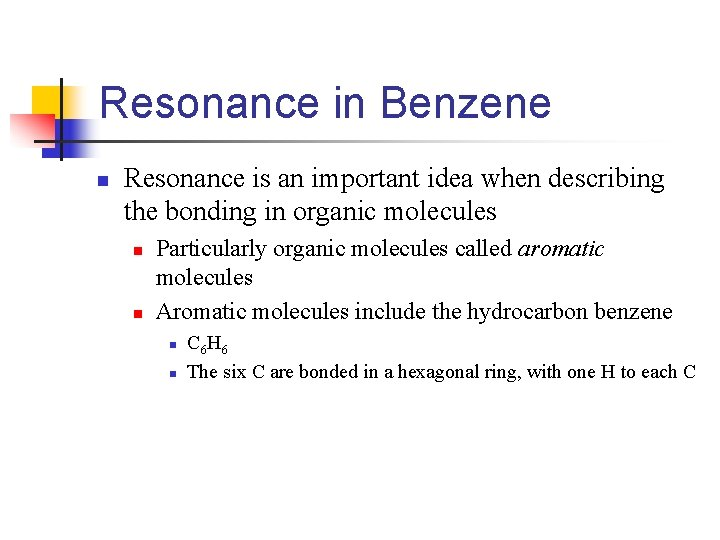Resonance in Benzene n Resonance is an important idea when describing the bonding in organic molecules n n Particularly organic molecules called aromatic molecules Aromatic molecules include the hydrocarbon benzene n n C 6 H 6 The six C are bonded in a hexagonal ring, with one H to each C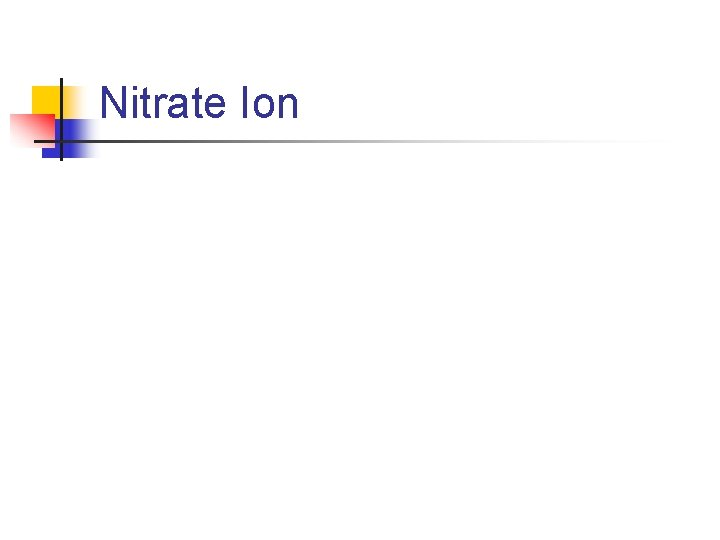Nitrate Ion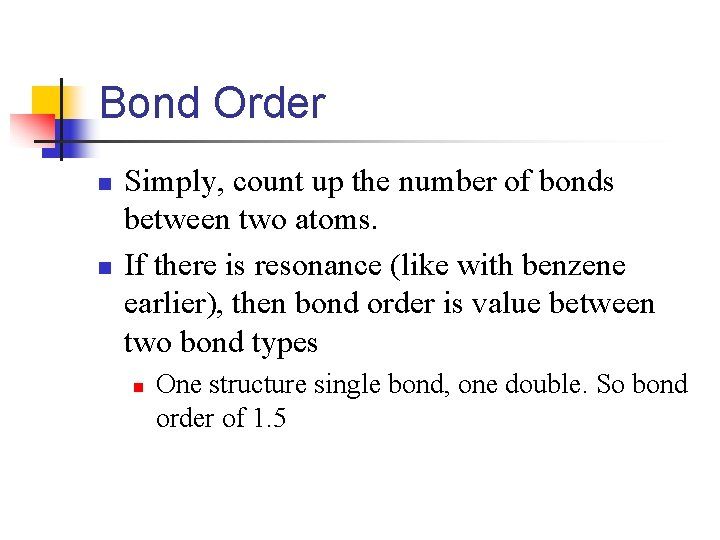Bond Order n n Simply, count up the number of bonds between two atoms. If there is resonance (like with benzene earlier), then bond order is value between two bond types n One structure single bond, one double. So bond order of 1. 5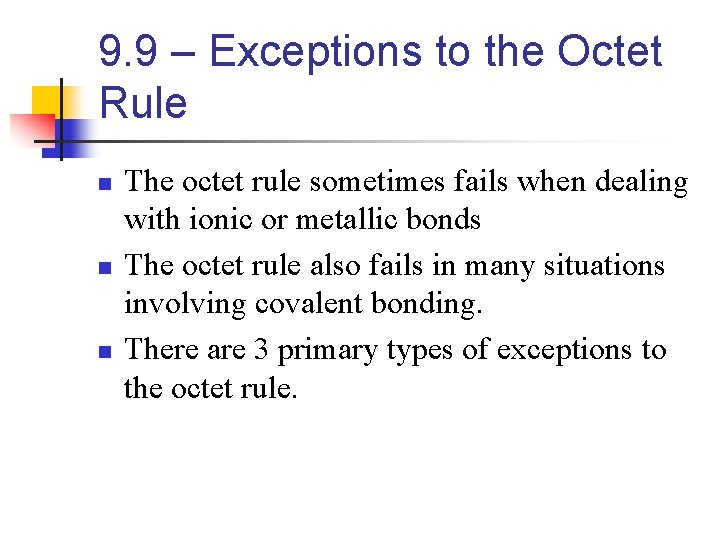9. 9 – Exceptions to the Octet Rule n n n The octet rule sometimes fails when dealing with ionic or metallic bonds The octet rule also fails in many situations involving covalent bonding. There are 3 primary types of exceptions to the octet rule.The 3 Exceptions!!!!!! n 1. 2. 3. Molecules and polyatomic ions… … with an odd number of electrons … where an atom has less than an octet of valence electrons … where an atom has more than an octet of valence electrons No longer tested on AP examOdd Number of Electrons n n n In most molecules and polyatomic ions, the number of valence electrons is even But if there is an odd number, an octet around each atom can’t be achieved. This often leads to resonance structures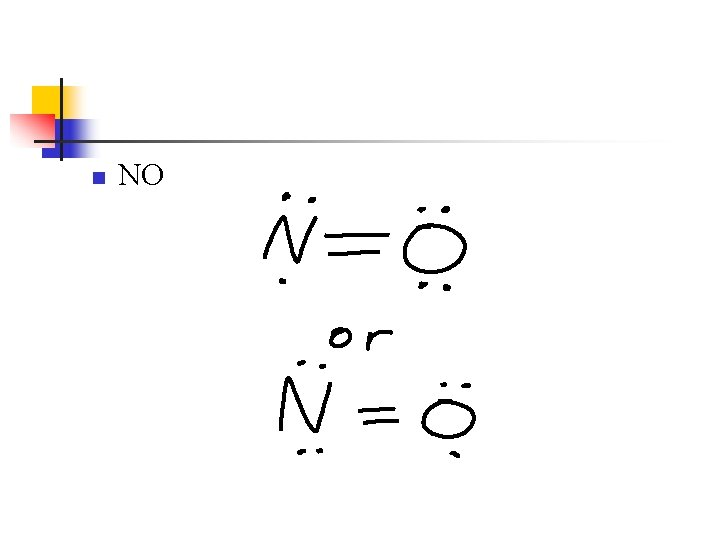n NOMore Than An Octet of Valence Electrons n n This is the largest class of exceptions This can only occur with elements in the 3 rd period or higher n n This is because they can use their empty d orbitals to place additional electrons 2 nd period and lower doesn’t have d orbitals, so must follow octet ruleBut wait, there’s more! n Atomic size influences if a molecule or polyatomic ion will take more than 8 valence electrons n The larger the central atom, the more atoms can surround it. n n So the larger the central atom, the more likely it can have more than an octet Also more likely if the central atom is surrounded by small and electronegative atoms n Such as F, Cl, and On There are times when you CAN write an octet-following Lewis structure and a nonoctet following Lewis structure n n Maybe because the non-octet following structure has better formal charges In general, choose the structure that satisfies the octet rule if possible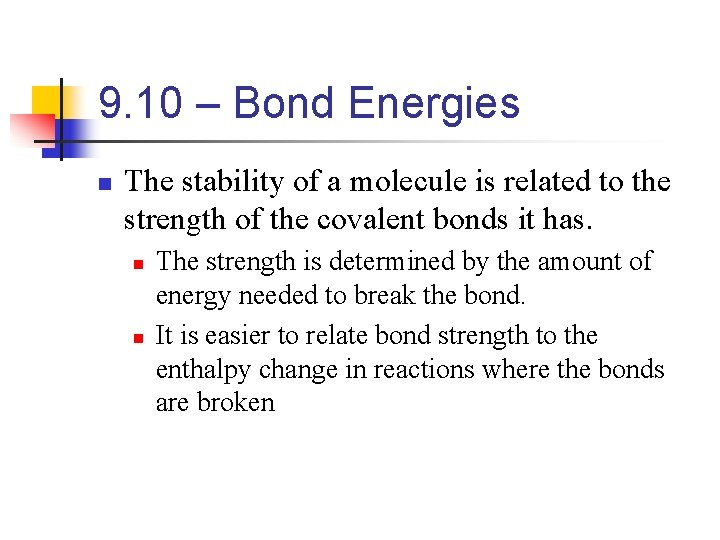9. 10 – Bond Energies n The stability of a molecule is related to the strength of the covalent bonds it has. n n The strength is determined by the amount of energy needed to break the bond. It is easier to relate bond strength to the enthalpy change in reactions where the bonds are brokenBond Enthalpies and Enthalpies of Reactions n We can use average bond enthalpies to estimate the enthalpies of reactions n n n Where bonds are broken and new bond are formed Lets us determine if a reaction will be endothermic or exothermic Even if we don’t know the ΔHºf for all the chemicals involved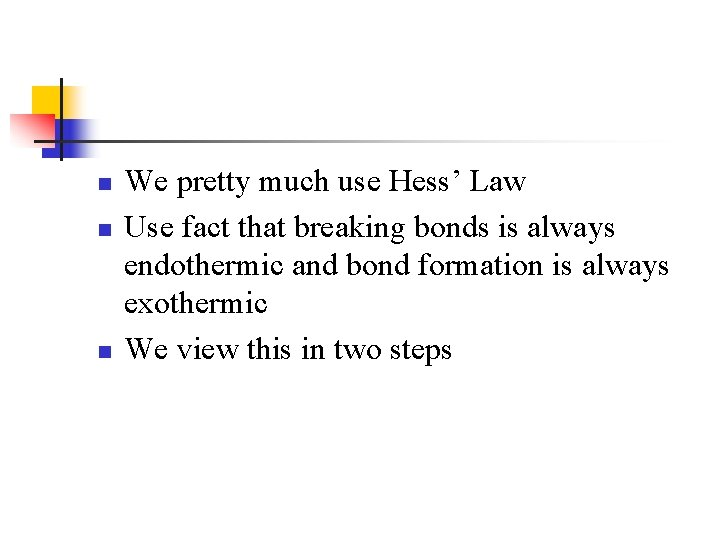n n n We pretty much use Hess’ Law Use fact that breaking bonds is always endothermic and bond formation is always exothermic We view this in two steps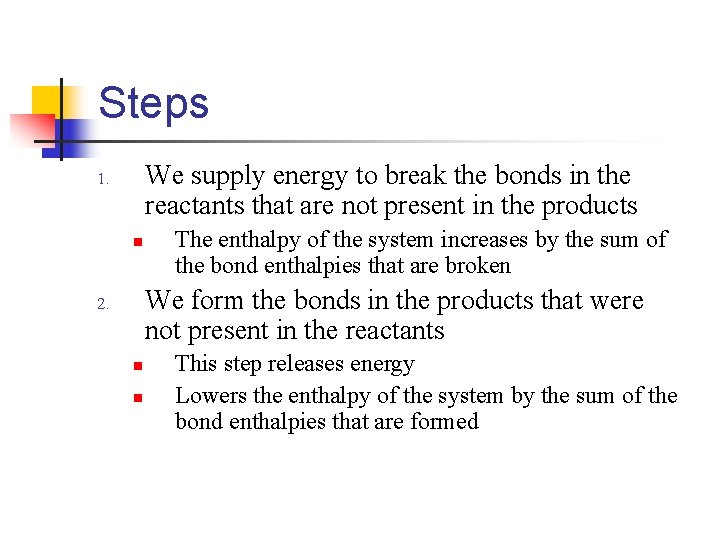Steps We supply energy to break the bonds in the reactants that are not present in the products 1. n The enthalpy of the system increases by the sum of the bond enthalpies that are broken We form the bonds in the products that were not present in the reactants 2. n n This step releases energy Lowers the enthalpy of the system by the sum of the bond enthalpies that are formed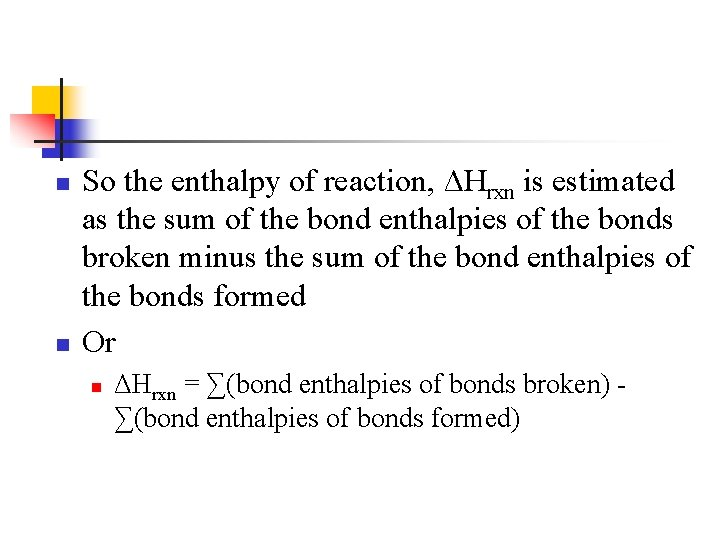n n So the enthalpy of reaction, ΔHrxn is estimated as the sum of the bond enthalpies of the bonds broken minus the sum of the bond enthalpies of the bonds formed Or n ΔHrxn = ∑(bond enthalpies of bonds broken) ∑(bond enthalpies of bonds formed)Example n n n Reaction between methane (CH 4) and chlorine (Cl 2) to produce methyl chloride (CH 3 Cl) and hydrogen chloride (HCl) H-CH 3(g) + Cl-Cl(g) Cl-CH 3(g) + HCl(g) ΔHrxn = ?n Bonds broken n n Bonds formed n n 1 mol C-H, 1 mol Cl-Cl 1 mol C-Cl, 1 mol H-Cl We use the equation previously given and the data on pg. 330 on table 8. 4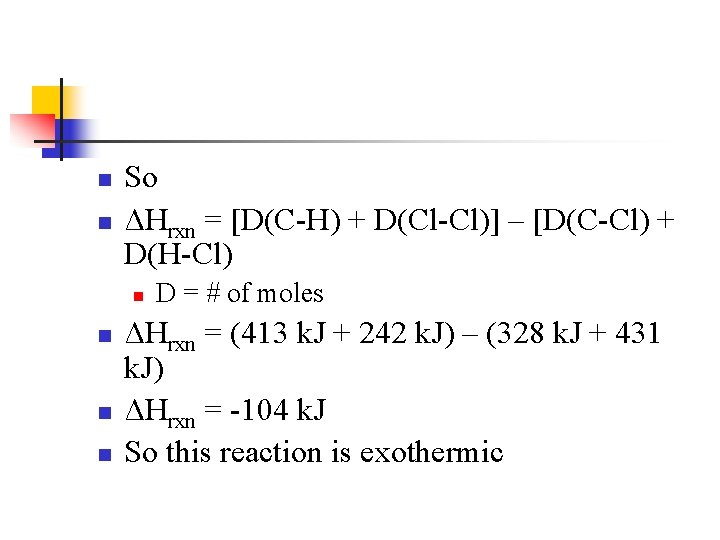n n So ΔHrxn = [D(C-H) + D(Cl-Cl)] – [D(C-Cl) + D(H-Cl) n n D = # of moles ΔHrxn = (413 k. J + 242 k. J) – (328 k. J + 431 k. J) ΔHrxn = -104 k. J So this reaction is exothermic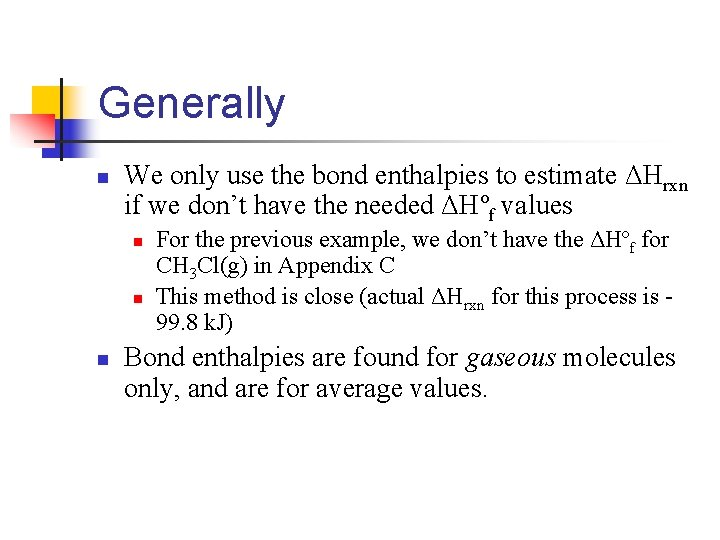Generally n We only use the bond enthalpies to estimate ΔHrxn if we don’t have the needed ΔHºf values n n n For the previous example, we don’t have the ΔHºf for CH 3 Cl(g) in Appendix C This method is close (actual ΔHrxn for this process is 99. 8 k. J) Bond enthalpies are found for gaseous molecules only, and are for average values.Example n What is the ΔH of this reaction?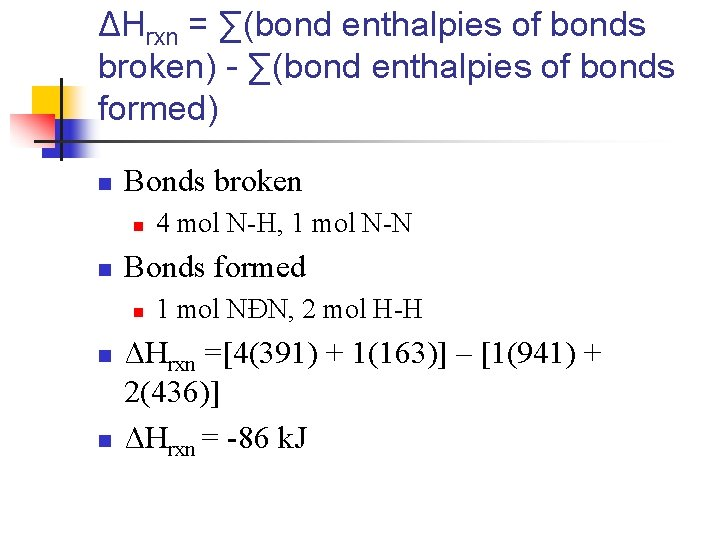ΔHrxn = ∑(bond enthalpies of bonds broken) - ∑(bond enthalpies of bonds formed) n Bonds broken n n Bonds formed n n n 4 mol N-H, 1 mol N-N 1 mol NÐN, 2 mol H-H ΔHrxn =[4(391) + 1(163)] – [1(941) + 2(436)] ΔHrxn = -86 k. J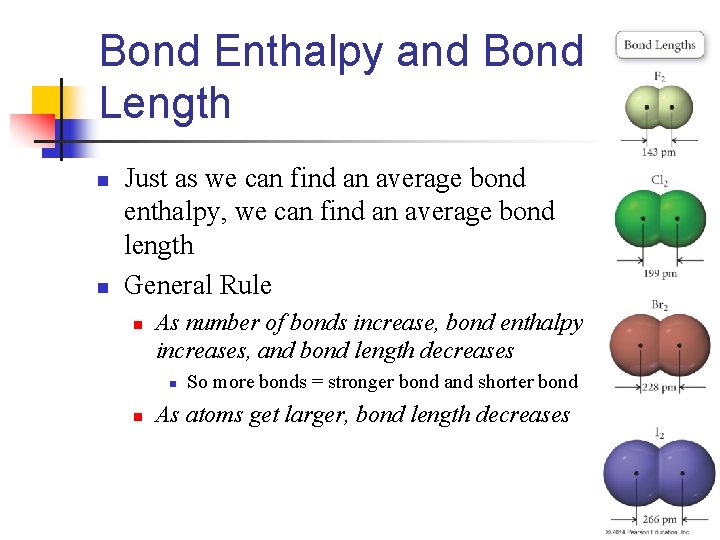Bond Enthalpy and Bond Length n n Just as we can find an average bond enthalpy, we can find an average bond length General Rule n As number of bonds increase, bond enthalpy increases, and bond length decreases n n So more bonds = stronger bond and shorter bond As atoms get larger, bond length decreases